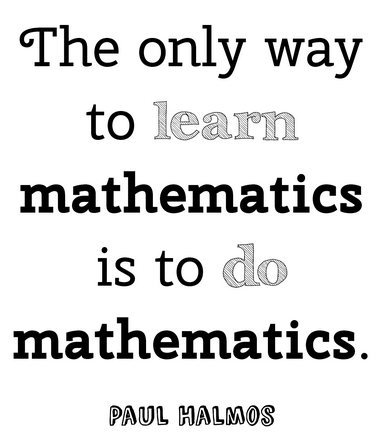Algebra Workshop Part 2

17 QuestionsSettingsWelcome to Algebra Workshop Part 2. If you are reading this, you scored 75% or higher on algebra Workshop Part 1. This means you are likely to place into algebra or advanced algebra when you take the OCCC Placement test. At the end of the following workshop, you will see recommendations to prepare you for either of these classes. Every question has links at the end of it, including the problem worked out step by step. Please take your time and use these links to your advantage. Remember, this could save you time and money.

• 1.
Candidate A received 2,347 votes in the last election, which was 38% of the total number of votes cast. How many votes were cast in the last election? Round your answer to the nearest whole number.
• A.

6176

• B.

892

• C.

89,186

• D.

8918

• 2.
What is the sum of
• A.
• B.
• C.
• D.
• 3.
Which is a factor of
• A.

(x+5)

• B.

(x-10)

• C.

(x+2)

• D.

(x-2)

• 4.
Simplify
• A.
• B.
• C.
• D.
• 5.
Simplify: =
• A.

-5/4

• B.

-3/4

• C.

0

• D.

-2

• 6.
Write the equation of the line in y=mx+b form with the slope -2 that passes through the point (-1,4)
• A.

Y=-2x+2

• B.

Y=2x-2

• C.

Y=4x-2

• D.

Y=-x-2

• 7.
Simplify:
• A.
• B.
• C.
• D.
• 8.
Simplify:
• A.
• B.
• C.
• D.
• 9.
Simplify:
• A.
• B.
• C.
• D.
• 10.
Simplify:
• A.
• B.
• C.
• D.
• 11.
Factor and solve.
• A.

(2,-5)

• B.

(-5,-2)

• C.

(5,2)

• D.

(-2,5)

• 12.
Solve.
• A.

8, -2

• B.

5, -5

• C.

8, -8

• D.

8, 2

• 13.
Simplify:
• A.
• B.
• C.
• D.
• 14.
Simplify:
• A.
• B.
• C.
• D.
• 15.
Simplify:
• A.
• B.
• C.

18

• D.
• 16.
Simplify:
• A.
• B.
• C.
• D.
• 17.
Solve.
• A.

2

• B.

-2

• C.

3

• D.

-3